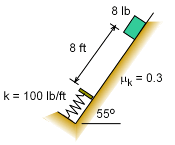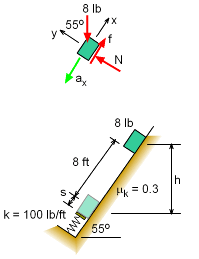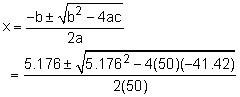Ch 3. Particle Energy Methods Multimedia Engineering Dynamics Work &Energy Conservative Forces Power & Efficiency
 Chapter - Particle - 1. General Motion 2. Force & Accel. 3. Energy 4. Momentum - Rigid Body - 5. General Motion 6. Force & Accel. 7. Energy 8. Momentum 9. 3-D Motion 10. Vibrations Appendix Basic Math Units Basic Equations Sections Search eBooks Dynamics Fluids Math Mechanics Statics Thermodynamics Author(s): Kurt Gramoll ©Kurt GramollDYNAMICS - EXAMPLEBox Slide with Sprint Example An 8 lb box slides down slope (kinetic coefficient of friction, μk = 0.1) and compresses a spring. How far is the spring compress before the box is pushed back up the slope? SolutionFBD and Spring Compression The problem involves both energy and forces. The friction force needs to be determined before energy methods can be applied. Find the normal and friction forces,      ΣFy = 0      N = 8 cos55 = 4.589 lb      f = μN = 0.3 (4.589) = 1.377 lb Energy balance      T1 +  U1-2 = T2      0 + (mgh - fd) = 0.5 k s2      8 (8 + s) sin55 - 1.377 (8 + s) = 0.5 (100) s2      52.43 + 6.553s - 11.01 - 1.377 s = 50 s2      50s2 - 5.176s - 41.42 = 0 Solving the quadratic equations = 0.05176 +/- 0.9116     (use positive answer) s = 0.9634 ft

Practice Homework and Test problems now available in the 'Eng Dynamics' mobile app
Includes over 400 problems with complete detailed solutions.
Available now at the Google Play Store and Apple App Store.